Home Practice
For learners and parents For teachers and schools
Textbooks
Full catalogue
Pricing SupportLog in

We think you are located in United States. Is this correct?

# 5.6 Snell's Law

## 5.6 Snell's Law (ESBN8)

Now that we know that the degree of bending, or the angle of refraction, is dependent on the refractive index of a medium, how do we calculate the angle of refraction? The answer to this question was discovered by a Dutch physicist called Willebrord Snell in 1621 and is now called Snell's Law or the Law of Refraction.

Snell's law

$\boxed{n_1 \sin \theta_1 = n_2 \sin \theta_2}$ where $\begin{array}{rl} n_1 &= \text{Refractive index of material 1} \\ n_2 &= \text{Refractive index of material 2} \\ \theta_1 &= \text{Angle of incidence} \\ \theta_2 &= \text{Angle of refraction} \\ \end{array}$

Remember that angles of incidence and refraction are measured from the normal, which is an imaginary line perpendicular to the surface.

Suppose we have two media with refractive indices $$n_1$$ and $$n_2$$. A light ray is incident on the surface between these materials with an angle of incidence $$\theta_1$$. The refracted ray that passes through the second medium will have an angle of refraction $$\theta_2$$.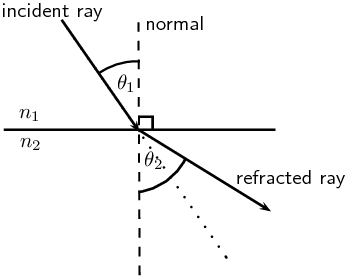Snell never published his discovery of the Law of Refraction. His work was actually published by another prominent physicist of the time, Christiaan Huygens, who gave credit to Snell.

A recommended project for formal assessment on verifying Snell's laws and determining the refractive index of an unknown transparent solid should be done. Learners will need a glass block, ray box, 0-360 protractor, a glass block, a transparent block of an unknown material and A4 paper. This project is given as two formal experiments, which learners can complete and then write up as a project. The project should include some background information on Snell's law, all the steps taken in the experiments, key experimental results and a conclusion about the experiment (including identification of the unknown solid from the refractive index).

## Verifying Snell's Law

### Aim

To verify Snell's law

### Apparatus

glass block, ray box, $$\text{360}$$$$\text{°}$$ protractor, 5 pieces of A4 paper, pencil, ruler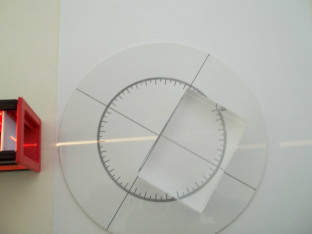### Method

This experiment will require you to follow the steps below 5 times (once for each piece of A4 paper).

1. Place the glass block in the middle of the A4 piece of paper so that its sides are parallel to each of the sides of the paper and draw around the block with a pencil to make its outline on the piece of paper.

2. Turn on the ray box and aim the light ray towards the glass block so that it makes an angle with the nearest surface of the block as shown in the picture. For each piece of paper, change the angle of the incoming ray.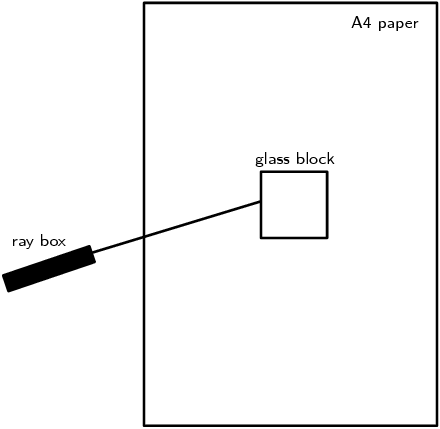3. You will now need to mark on the paper, the path of the incoming and outgoing light rays. Do this by first drawing a dot on the paper somewhere along the incoming light ray. Now draw a second dot on the paper at the point where the incoming light ray hits the surface of the block. Do the same thing for the outgoing light ray; mark the point where it leaves the block and some other point along its path.

4. Now switch off the ray box and remove the glass block from the paper. Use a ruler to join the dots of the incoming ray. Now join the dots of the outgoing ray. Lastly, draw a line which joins the point where the incoming ray hits the block and where the outgoing ray leaves the block. This is the path of the light ray through the glass.

5. The aim of this experiment is to verify Snell's law. i.e. $$n_1 \sin \theta_1 = n_2 \sin \theta_2$$. We know the refractive index of our two media:

For air, $$n_1 =\text{1,0}$$

For glass, $$n_2 =\text{1,5}$$

Now we need to measure the two angles, $$\theta_1$$ and $$\theta_2$$. To do this, we need to draw the normal to the surface where the light ray enters the block. Use the protractor to measure an angle of $$\text{90}$$$$\text{°}$$ to the entry surface and draw the normal. At this point, the drawing on your piece of paper should look something like the picture: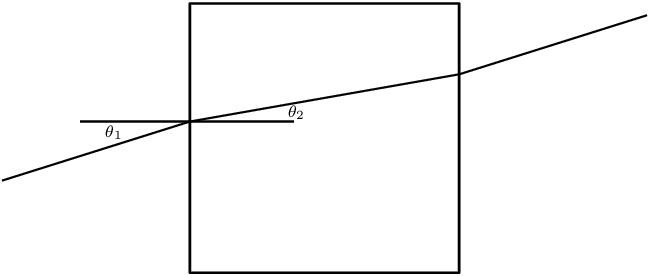6. Now measure $$\theta_1$$ and $$\theta_2$$ using the protractor. Enter the values you measured into a table which looks like:

 $$\theta_1$$ $$\theta_2$$ $$n_1 \sin \theta_1$$ $$n_2 \sin \theta_2$$

### Discussion

Have a look at your completed table. You should have 5 rows filled in, one for each of your pieces of A4 paper. For each row, what do you notice about the values in the last two columns? Do your values agree with what Snell's law predicts?

## Using Snell's law to determine the refractive index of an unknown material

### Aim

To determine the refractive index of an unknown material

### Apparatus

ray box, $$\text{360}$$$$\text{°}$$ protractor, 5 pieces of A4 paper, a block of unknown transparent material, pencil, ruler

### Method

This experiment will require you to follow the steps below at least 5 times. The steps are the same as you followed in the previous experiment.

1. Place the block in the middle of the A4 piece of paper so that its sides are parallel to each of the sides of the paper and draw around the block with a pencil to make its outline on the piece of paper.

2. Turn on the ray box and aim the light ray towards the block so that it makes an angle with the nearest surface of the block. For each piece of paper, change the angle of the incoming ray.

3. You will now need to mark on the paper, the path of the incoming and outgoing light rays. Do this by first drawing a dot on the paper somewhere along the incoming light ray. Now draw a second dot on the paper at the point where the incoming light ray hits the surface of the block. Do the same thing for the outgoing light ray; mark the point where it leaves the block and some other point along its path.

4. Now switch off the ray box and remove the block from the paper. Use a ruler to join the dots of the incoming ray. Now join the dots of the outgoing ray. Lastly, draw a line which joins the point where the incoming ray hits the block and where the outgoing ray leaves the block. This is the path of the light ray through the block.

5. The aim of this experiment is to determine the refractive index $$n_2$$ of the unknown material using Snell's law which states $$n_1 \sin \theta_1 = n_2 \sin \theta_2$$. We know that for air $$n_1$$ = $$\text{1,0}$$ and we can measure the angle of incidence, $$\theta_1$$ and the angle of refraction, $$\theta_2$$. Then we can solve the equation for the unknown, $$n_2$$.

To measure $$\theta_1$$ and $$\theta_2$$, we need to draw the normal to the surface where the light ray enters the block. Use the protractor to measure an angle of $$\text{90}$$$$\text{°}$$ to the entry surface and draw the normal. Now use the protractor to measure $$\theta_1$$ and $$\theta_2$$.

6. Enter the values you measured into a table which looks like the one below and calculate the resulting value for $$n_2$$.

 $$\theta_1$$ $$\theta_2$$ $$n_2 = \frac{n_1 \sin \theta_1}{\sin \theta_2}$$

### Question

What do you notice about all your values in the last column of the table?

### Discussion

You should have noticed that the values in the last column of the table are similar but not identical. This is due to measurement errors when you measured the angles of incidence and angles of refraction. These sorts of errors are common in all physics experiments and lead to a measure of uncertainty in the final extracted value. However, since we did the same experiment 5 times, we can average the 5 independent measurements of $$n_2$$ to get a good approximation to the real value for our unknown material.

If $n_2 > n_1$ then from Snell's Law, $\sin \theta_1 > \sin \theta_2$

For angles smaller than $$\text{90}$$$$\text{°}$$, $$\sin{\theta}$$ increases as $$\theta$$ increases. Therefore, $\theta_1 > \theta_2.$ This means that the angle of incidence is greater than the angle of refraction and the light ray is bent toward the normal.

Similarly, if $n_2 < n_1$ then from Snell's Law, $\sin \theta_1 < \sin \theta_2.$ For angles smaller than $$\text{90}$$$$\text{°}$$, $$\sin{\theta}$$ increases as $$\theta$$ increases. Therefore, $\theta_1 < \theta_2.$ This means that the angle of incidence is less than the angle of refraction and the light ray is bent away from the normal.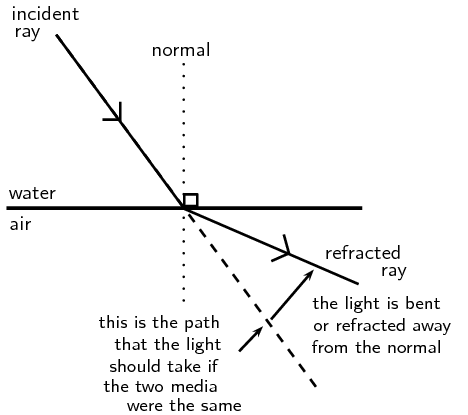Figure 5.12: Light is moving from a medium with a higher refractive index to one with a lower refractive index. Light is refracted away from the normal.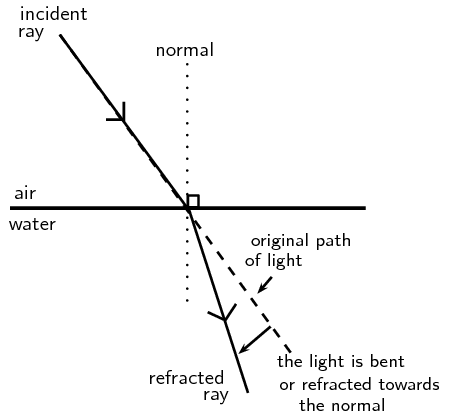Figure 5.13: Light is moving from a medium with a lower refractive index to a medium with a higher refractive index. Light is refracted towards the normal.

What happens to a ray that lies along the normal line? In this case, the angle of incidence is $$\text{0}$$$$\text{°}$$ and \begin{align*} \sin \theta_2 &= \frac{n_1}{n_2} \sin \theta_1 \\ &= \text{0} \\ \therefore \theta_2 &= \text{0}. \end{align*}

This shows that if the light ray is incident at $$\text{0}$$$$\text{°}$$, then the angle of refraction is also $$\text{0}$$$$\text{°}$$. The direction of the light ray is unchanged, however, the speed of the light will change as it moves into the new medium. Therefore refraction still occurs although it will not be easily observed.

Let's use an everyday example that you can more easily imagine. Imagine you are pushing a lawnmower or a cart through short grass. As long as the grass is pretty much the same length everywhere, it is easy to keep the mower or cart going in a straight line. However, if the wheels on one side of the mower or cart enter an area of grass that is longer than the grass on the other side, that side of the mower will move more slowly since it is harder to push the wheels through the longer grass. The result is that the mower will start to turn inwards, towards the longer grass side as you can see in the picture. This is similar to light which passes through a medium and then enters a new medium with a higher refractive index or higher optical density. The light will change direction towards the normal, just like the mower in the longer grass. The opposite happens when the mower moves from an area of longer grass into an area with shorter grass. The side of the mower in the shorter grass will move faster and the mower will turn outwards, just like a light ray moving from a medium with high refractive index into a medium with low refractive index moves away from the normal.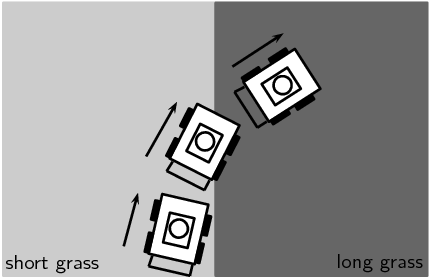Figure 5.14: The lawnmower is moving from an area of short grass into an area of longer grass. When it reaches the boundary between the areas, the wheels which are in the long grass move slower than the wheels which are still in the short grass, causing the lawnmower to change direction and its path to bend inwards.

## Worked example 2: Using Snell's Law

Light is refracted at the boundary between water and an unknown medium. If the angle of incidence is $$\text{25}$$$$\text{°}$$, and the angle of refraction is $$\text{20,6}$$$$\text{°}$$, calculate the refractive index of the unknown medium and use Table 5.1 to identify the material.

### Determine what is given and what is being asked

The angle of incidence $$\theta_{1}=$$$$\text{25}$$$$\text{°}$$

The angle of refraction $$\theta_{2}=$$$$\text{20,6}$$$$\text{°}$$

We can look up the refractive index for water in Table 5.1: $$n_{1} =\text{1,333}$$

We need to calculate the refractive index for the unknown medium and identify it.

### Determine how to approach the problem

We can use Snell's law to calculate the unknown refractive index, $$n_{2}$$

According to Snell's Law: \begin{align*} n_1 \sin \theta_1 &= n_2 \sin \theta_2 \\ n_2 &= \frac{n_1 \sin \theta_1}{\sin \theta_2} \\ n_2 &= \frac{\text{1,333} \sin \text{25}\text{°}}{\sin \text{20,6}\text{°}} \\ n_2 &= \text{1,6} \end{align*}

### Identify the unknown medium

According to Table 5.1, typical glass has a refractive index between $$\text{1,5}$$ to $$\text{1,9}$$. Therefore the unknown medium is typical glass.

## Worked example 3: Using Snell's law

A light ray with an angle of incidence of $$\text{35}$$$$\text{°}$$ passes from water to air. Find the angle of refraction using Snell's Law and Table 5.1. Discuss the meaning of your answer.

### Determine the refractive indices of water and air

From Table 5.1, the refractive index is $$\text{1,333}$$ for water and about $$\text{1}$$ for air. We know the angle of incidence, so we are ready to use Snell's Law.

### Substitute values

According to Snell's Law: \begin{align*} n_1 \sin \theta_1 &= n_2 \sin \theta_2 \\ \text{1,33} \sin \text{35}\text{°} &= \text{1} \sin \theta_2 \\ \sin \theta_2 &= \text{0,763} \\ \theta_2 &= \text{49,7}\text{°} \text{ or } \text{130,3}\text{°} \end{align*}

Since $$\text{130,3}$$$$\text{°}$$ is larger than $$\text{90}$$$$\text{°}$$, the solution is: $\theta_2 = \text{49,7}\text{°}$

The light ray passes from a medium of high refractive index to one of low refractive index. Therefore, the light ray is bent away from the normal.

## Worked example 4: Using Snell's Law

A light ray passes from water to diamond with an angle of incidence of $$\text{75}$$$$\text{°}$$. Calculate the angle of refraction. Discuss the meaning of your answer.

### Determine the refractive indices of water and air

From Table 5.1, the refractive index is $$\text{1,333}$$ for water and $$\text{2,42}$$ for diamond. We know the angle of incidence, so we are ready to use Snell's Law.

### Substitute values and solve

According to Snell's Law: \begin{align*} n_1 \sin \theta_1 &= n_2 \sin \theta_2 \\ \text{1,33} \sin \text{75}\text{°} &= \text{2,42} \sin \theta_2 \\ \sin{\theta_2} &= \text{0,531} \\ \theta_2 & = \text{32,1}\text{°} \end{align*}

The light ray passes from a medium of low refractive index to one of high refractive index. Therefore, the light ray is bent towards the normal.

temp text

## Snell's Law

Textbook Exercise 5.4

State Snell's Law.

Snell's law states that the angle of incidence times the refractive index of medium 1 is equal to the angle of refraction times the refractive index of medium 2. Mathematically this is: $$n_{1} \sin \theta_{1} = n_{2} \sin \theta_{2}$$

Light travels from a region of glass into a region of glycerine, making an angle of incidence of $$\text{40}$$$$\text{°}$$.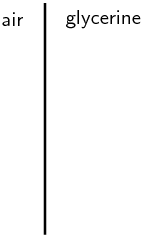Draw the incident and refracted light rays on the diagram and label the angles of incidence and refraction.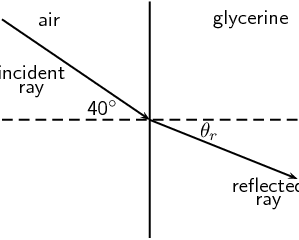Calculate the angle of refraction.

The refractive index of air is $$\text{1}$$ and the refractive index of glycerine is $$\text{1,4729}$$.

\begin{align*} n_1 \sin \theta_1 &= n_2 \sin \theta_2 \\ \text{1} \sin \text{40}\text{°} &= \text{1,4729} \sin \theta_2 \\ \sin{\theta_2} &= \text{0,436} \\ \theta_2 & = \text{25,88}\text{°} \end{align*}

A ray of light travels from silicon to water. If the ray of light in the water makes an angle of $$\text{69}$$$$\text{°}$$ to the normal to the surface, what is the angle of incidence in the silicon?

The refractive index of silicon is $$\text{4,01}$$ and the refractive index of water is $$\text{1,333}$$.

\begin{align*} n_1 \sin \theta_1 &= n_2 \sin \theta_2 \\ \text{1,333} \sin \text{69}\text{°} &= \text{4,01} \sin \theta_2 \\ \sin{\theta_2} &= \text{0,310} \\ \theta_2 & = \text{18,08}\text{°} \end{align*}

Light travels from a medium with $$n = \text{1,25}$$ into a medium of $$n = \text{1,34}$$, at an angle of $$\text{27}$$$$\text{°}$$ from the normal.

What happens to the speed of the light? Does it increase, decrease, or remain the same?

The speed of light decreases as it enters the second medium.

What happens to the wavelength of the light? Does it increase, decrease, or remain the same?

The wavelength of the light remains the same. Wavelength is related to frequency and the frequency of light does not change as it moves from one medium to another.

Does the light bend towards the normal, away from the normal, or not at all?

Towards the normal.

Light travels from a medium with $$n = \text{1,63}$$ into a medium of $$n = \text{1,42}$$.

What happens to the speed of the light? Does it increase, decrease, or remain the same?

The speed of light increases as it enters the second medium.

What happens to the wavelength of the light? Does it increase, decrease, or remain the same?

The wavelength of the light remains the same. Wavelength is related to frequency and the frequency of light does not change as it moves from one medium to another.

Does the light bend towards the normal, away from the normal, or not at all?

Away from the normal

Light is incident on a rectangular glass prism. The prism is surrounded by air. The angle of incidence is $$\text{23}$$$$\text{°}$$. Calculate the angle of reflection and the angle of refraction.

The angle of reflection is the same as the angle of incidence: $$\text{23}$$$$\text{°}$$.

To find the angle of refraction we need the indices of refraction for glass and air. The index of refraction for air is $$\text{1}$$ and for the prism it is $$\text{1,5}$$ - $$\text{1,9}$$ (we assume that the prism is made of typical glass). Since typical glass has a range of refractive indices we will calculate the maximum and minimum possible angles of refraction.

The maximum possible angle of refraction is:

\begin{align*} n_1 \sin \theta_1 &= n_2 \sin \theta_2 \\ \text{1} \sin \text{23}\text{°} &= \text{1,5} \sin \theta_2 \\ \sin{\theta_2} &= \text{0,2604} \\ \theta_2 & = \text{15,10}\text{°} \end{align*}

The minimum possible angle of refraction is:

\begin{align*} n_1 \sin \theta_1 &= n_2 \sin \theta_2 \\ \text{1} \sin \text{23}\text{°} &= \text{1,9} \sin \theta_2 \\ \sin{\theta_2} &= \text{0,2056} \\ \theta_2 & = \text{11,87}\text{°} \end{align*}

Light is refracted at the interface between air and an unknown medium. If the angle of incidence is $$\text{53}$$$$\text{°}$$ and the angle of refraction is $$\text{37}$$$$\text{°}$$, calculate the refractive index of the unknown, second medium.

The index of refraction for air is $$\text{1}$$. The refractive index of the unknown medium is:

\begin{align*} n_1 \sin \theta_1 &= n_2 \sin \theta_2 \\ \text{1} \sin \text{53}\text{°} &= n_{2} \sin \text{37}\text{°} \\ n_{2} &= \text{1,327} \end{align*}

Light is refracted at the interface between a medium of refractive index $$\text{1,5}$$ and a second medium of refractive index $$\text{2,1}$$. If the angle of incidence is $$\text{45}$$$$\text{°}$$, calculate the angle of refraction.

The angle of refraction is:

\begin{align*} n_1 \sin \theta_1 &= n_2 \sin \theta_2 \\ \text{1,5} \sin \text{45}\text{°} &= \text{2,1} \sin \theta_{2} \\ \sin \theta_{2} &= \text{0,5051} \\ \theta_{2} & = \text{30,34}\text{°} \end{align*}

A ray of light strikes the interface between air and diamond. If the incident ray makes an angle of $$\text{30}$$$$\text{°}$$ with the interface, calculate the angle made by the refracted ray with the interface.

The refractive index of air is 1 and the refractive index of diamond is $$\text{2,419}$$. The angle of refraction is:

\begin{align*} n_1 \sin \theta_1 &= n_2 \sin \theta_2 \\ \text{1} \sin \text{30}\text{°} &= \text{2,419} \sin \theta_{2} \\ \sin \theta_{2} &= \text{0,20669} \\ \theta_{2} & = \text{11,93}\text{°} \end{align*}

This is the angle between the normal and the refracted ray. The question actually wants the angle between the refracted ray and the interface. This angle is:

\begin{align*} \text{90}\text{°} - \text{11,93}\text{°} = \text{78,07}\text{°} \end{align*}

The angles of incidence and refraction were measured in five unknown media and recorded in the table below. Use your knowledge about Snell's Law to identify each of the unknown media A–E. Use Table 5.1 to help you.

 Medium 1 $$n_1$$ $$\theta_1$$ $$\theta_2$$ $$n_2$$ Unknown Medium Air $$\text{1,0002926}$$ $$\text{38}$$ $$\text{27}$$ ? A Air $$\text{1,0002926}$$ $$\text{65}$$ $$\text{38,4}$$ ? B Vacuum $$\text{1}$$ $$\text{44}$$ $$\text{16,7}$$ ? C Air $$\text{1,0002926}$$ $$\text{15}$$ $$\text{6,9}$$ ? D Vacuum $$\text{1}$$ $$\text{20}$$ $$\text{13,3}$$ ? E

For substance A, the refractive index is:

\begin{align*} n_1 \sin \theta_1 &= n_2 \sin \theta_2 \\ \text{1,0002926} \sin \text{38}\text{°} &= n_{2} \sin \text{27}\text{°} \\ n_{2} & = \text{1,36} \end{align*}

Substance A is acetone.

For substance B, the refractive index is:

\begin{align*} n_1 \sin \theta_1 &= n_2 \sin \theta_2 \\ \text{1,0002926} \sin \text{65}\text{°} &= n_{2} \sin \text{38,4}\text{°} \\ n_{2} & = \text{1,459} \end{align*}

Substance B is fused quartz.

For substance C, the refractive index is:

\begin{align*} n_1 \sin \theta_1 &= n_2 \sin \theta_2 \\ \text{1} \sin \text{44}\text{°} &= n_{2} \sin \text{16,7}\text{°} \\ n_{2} & = \text{2,419} \end{align*}

Substance C is diamond.

For substance D, the refractive index is:

\begin{align*} n_1 \sin \theta_1 &= n_2 \sin \theta_2 \\ \text{1,0002926} \sin \text{15}\text{°} &= n_{2} \sin \text{6,9}\text{°} \\ n_{2} & = \text{2,15} \end{align*}

Substance D is cubic zirconia.

For substance E, the refractive index is:

\begin{align*} n_1 \sin \theta_1 &= n_2 \sin \theta_2 \\ \text{1} \sin \text{20}\text{°} &= n_{2} \sin \text{13,3}\text{°} \\ n_{2} & = \text{1,49} \end{align*}

Substance E is an $$\text{80}\%$$ sugar solution.

Zingi and Tumi performed an investigation to identify an unknown liquid. They shone a ray of light into the unknown liquid, varying the angle of incidence and recording the angle of refraction. Their results are recorded in the following table:

 Angle of incidence Angle of refraction $$\text{0,0}$$$$\text{°}$$ $$\text{0,00}$$$$\text{°}$$ $$\text{5,0}$$$$\text{°}$$ $$\text{3,76}$$$$\text{°}$$ $$\text{10,0}$$$$\text{°}$$ $$\text{7,50}$$$$\text{°}$$ $$\text{15,0}$$$$\text{°}$$ $$\text{11,2}$$$$\text{°}$$ $$\text{20,0}$$$$\text{°}$$ $$\text{14,9}$$$$\text{°}$$ $$\text{25,0}$$$$\text{°}$$ $$\text{18,5}$$$$\text{°}$$ $$\text{30,0}$$$$\text{°}$$ $$\text{22,1}$$$$\text{°}$$ $$\text{35,0}$$$$\text{°}$$ $$\text{25,5}$$$$\text{°}$$ $$\text{40,0}$$$$\text{°}$$ $$\text{28,9}$$$$\text{°}$$ $$\text{45,0}$$$$\text{°}$$ $$\text{32,1}$$$$\text{°}$$ $$\text{50,0}$$$$\text{°}$$ $$\text{35,2}$$$$\text{°}$$ $$\text{55,0}$$$$\text{°}$$ $$\text{38,0}$$$$\text{°}$$ $$\text{60,0}$$$$\text{°}$$ $$\text{40,6}$$$$\text{°}$$ $$\text{65,0}$$$$\text{°}$$ $$\text{43,0}$$$$\text{°}$$ $$\text{70,0}$$$$\text{°}$$ ? $$\text{75,0}$$$$\text{°}$$ ? $$\text{80,0}$$$$\text{°}$$ ? $$\text{85,0}$$$$\text{°}$$ ?

Write down an aim for the investigation.

To use refractive indices and Snell's law to determine an unknown substance.

Make a list of all the apparatus they used.

Protractor, ruler, ray box, paper, pencils, beakers.

Identify the unknown liquid.

We can use any of the pairs of data to find the refractive index of the unknown substance. We will use the second pair of readings.

\begin{align*} n_1 \sin \theta_1 &= n_2 \sin \theta_2 \\ \text{1} \sin \text{5}\text{°} &= n_{2} \sin \text{3,76}\text{°} \\ n_{2} & = \text{1,329} \end{align*}

The substance is water.

Predict what the angle of refraction will be for $$\text{70}$$$$\text{°}$$, $$\text{75}$$$$\text{°}$$, $$\text{80}$$$$\text{°}$$ and $$\text{85}$$$$\text{°}$$.

The angle of refraction at $$\text{70}$$$$\text{°}$$ is:

\begin{align*} n_1 \sin \theta_1 &= n_2 \sin \theta_2 \\ \text{1} \sin \text{70}\text{°} &= \text{1,329} \sin \theta_{2} \\ \sin \theta_{2} & = \text{0,707} \\ n_{2} & = \text{45,0}\text{°} \end{align*}

The angle of refraction at $$\text{75}$$$$\text{°}$$ is:

\begin{align*} n_1 \sin \theta_1 &= n_2 \sin \theta_2 \\ \text{1} \sin \text{75}\text{°} &= \text{1,329} \sin \theta_{2} \\ \sin \theta_{2} & = \text{0,7268} \\ n_{2} & = \text{46,6}\text{°} \end{align*}

The angle of refraction at $$\text{80}$$$$\text{°}$$ is:

\begin{align*} n_1 \sin \theta_1 &= n_2 \sin \theta_2 \\ \text{1} \sin \text{80}\text{°} &= \text{1,329} \sin \theta_{2} \\ \sin \theta_{2} & = \text{0,741} \\ n_{2} & = \text{47,8}\text{°} \end{align*}

The angle of refraction at $$\text{85}$$$$\text{°}$$ is:

\begin{align*} n_1 \sin \theta_1 &= n_2 \sin \theta_2 \\ \text{1} \sin \text{85}\text{°} &= \text{1,329} \sin \theta_{2} \\ \sin \theta_{2} & = \text{0,75} \\ n_{2} & = \text{48,55}\text{°} \end{align*}